The Sundial Primer created by Carl SabanskiThe Sundial Primer Index
 Cycloid Polar Sundial Cycloid Polar Sundial:  a variation of the standard polar sundial in which the gnomon has a cycloid shape, with the result that the hour lines are equally spaced. Polar Sundial: is a sundial in which the dial plate is set along the East-West direction and inclines so that it is parallel with the polar axis. The standard polar pointing gnomon is thus also parallel to the dial plate. Latitude: is the angular position of a place north or south of the equator. Positive values in the Northern hemisphere, negative in the Southern hemisphere. Style Height / Style Angle (SH): of a polar style is the angle that the style makes with the sub-style line. Sub-Style (line): the line lying in the dial plane which is perpendicularly below (or behind for a vertical dial) the style. Hour Line: the line on a dial plate indicating the shadow position at a particular time (includes fractional as well as whole hours). Hour Angle (h, HA): the angle corresponding to the sun's position around its daily (apparent) orbit. Measured westward from local noon, it increases at a rate of 15° per hour. Thus 3 pm (Local Apparent Time) is 45° and 9 am is -45° Before we can design a cycloid polar sundial it would be good to know just what is a cycloid and how to draw one.  According to Webster:cycloid: the curve described by a point on the circumference of a circle that rolls, without slipping, along a straight line on a single plane.Figure 1 illustrates a cycloid and how it is created. The figure is of a cycloid with its vertex at the origin O, which is the type that is of interest for this particular dial. Just imagine the circle rolling on the horizontal dashed line. A single point on the circle will move from A to B to C and trace out the curve shown. Do the same in the other direction and the cycloid is created.Figure 1: CycloidThe mathematical equations that describe the x and y co-ordinates of the cycloid are as follows:x = r (f + sin f)y = r (1 - cos f)where r is the radius of the circle and f is the angle of rotation of the circle, which varies from -180° to 180°. f must be expressed in radians where:f in radians = (f in degrees/180) pFrom Figure 1 it can be seen that the height of the cycloid varies from 0 to the 2r or the diameter of the circle. The length L of the cycloid is 2pr or the circumference of the circle. This information is useful when determining the size of the sundial to be designed.The cycloid polar sundial is universal and can be used at any latitude. The hour lines are parallel to each other and are symmetrical with respect to the centre line that represents both 6 am and 6 pm. The gnomon, which takes the shape of a cycloid, is positioned perpendicular to this line. The dial plate lies parallel to the Earth's polar axis, i.e. it is tilted to an angle equal to the latitude where it is located. This is the same for a polar sundial. The hour lines at the far ends of the dial plate represent noon. What is unique about this dial is that the hour lines are equally spaced. Figure 2 attempts to illustrate why this is so.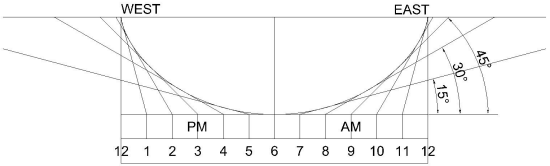Figure 2: Cycloid Polar Dial Hour LinesAs the dial plate lies parallel to Earth's polar axis the hour plane increases, relative to the dial plate, by 15° per hour. What is unique about the cycloid shape of the style is that the hour plane is tangent to only one point on this surface at any given time. This can be seen by the lines shown in Figure 2. When these planes are extended to the the surface of the dial plate to establish the hour lines it can also be seen that these lines are separated by an equal distance.The hour lines on the dial plate can be laid out to represent the hour angles in the range of ±90°. The negative hour angles are morning hours and the positive are the afternoon. The distance to each of the hour lines from the centre of the dial plate can be calculated as follows.X = r (h / 90 ± 1) p where h is the hour angle, in degrees, given by:h = (T24 - 12) * 15°and T24 is the time in 24-hour clock notation (hours after midnight) in decimal hours.Note that 1 is added for the morning hours and subtracted for the afternoon hours. This results in X varying from 0 to rp in the morning and from -rp to 0 in the afternoon.It is also possible to calculate the the x and y co-ordinates of the cycloid based upon the hour angle h. Figures 1 and 2 show that when a period of 1 hour has passed and the hour angle has changed by 15° the circle will have rotated through an angle of 30°, i.e. f = 2h ± 180° where 180° is for the morning hours and -180° is for the afternoon hours. Note that the the hour angles pass through the range of ±90° while the circle revolves through the range of ±180°. The equations can now be written as follows:x = r {(h / 90 ± 1) p - sin 2h}y = r (1 + cos 2h)The only factor that will change the cycloid is the variable r and this is just a scaling factor. When one dial is drawn then every other possible dial can be obtained by scaling the cycloid and dial plate.Figure 3 illustrates the completed dial template with 5 minute intervals. Click here to download a PDF file of the template. This template can be printed at any scale. The gnomon can also be modified to make it visually more interesting as long as the cycloid curve is not changed. Click here to download a DXF file of the template.The cycloid template was built using 1/4° movements of the circle turning to calculate the x and y co-ordinates. That's about 1444 sets over 360°. Click here to download a spreadsheet that will automatically calculate the cycloid for as small a movement of the circle as you want. You will end up with a lot of numbers that you then have to do something with. This is what I did to get a drawing of the cycloid using CAD software. I moved the two columns of numbers for the x and y co-ordinates to a new spreadsheet and saved this sheet as a comma delimited file, a *.csv in my case. I then opened this file in a text editor and made sure that there was a column of two numbers separated by commas with a return after each set of numbers. I copied everything into the Clipboard. I started a new drawing in the CAD package and initiated the "line" draw command. There is a command line available where I can type in a set of x and y co-ordinates for the first point of the line. I then select "Enter" and am prompted for the next set of co-ordinates and so on. I pasted the entire column of co-ordinates in this command line and the CAD software treated this as a series of line segments, which it drew. I then converted all these lines into a polyline that then allowed me to do a curve fit of all the small straight line segments. If your drawing software has a command line for entering co-ordinates to draw a line, this procedure may work.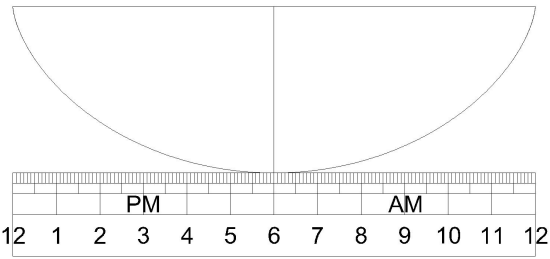Figure 3: Cycloid Polar Sundial TemplateThe cycloid gnomon only contacts the dial plate at one point and so could be difficult to attach. The dial can be split at the centre and a section inserted to make attachment easier. The gnomon can also be any thickness. A thick gnomon will provide a straight line shadow at the point where the time is indicated. Figure 4 illustrates a split dial.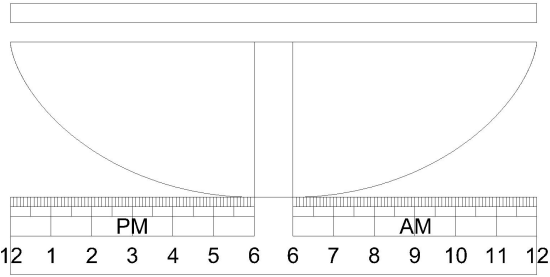Figure 4: Split Cycloid Polar SundialThe hour lines for this dial can be corrected for longitude.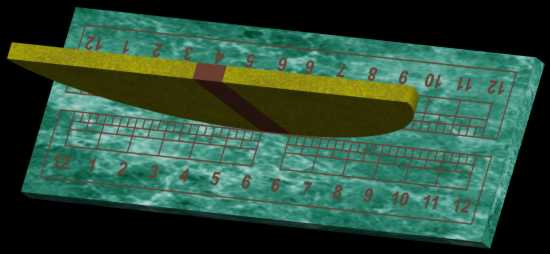For an image complete with shadow click here.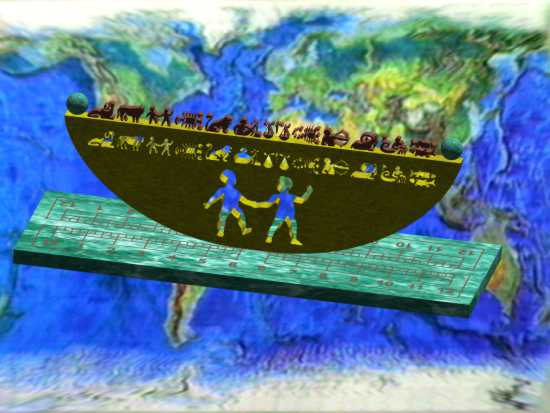The cycloid gnomon lies in the equatorial plane and is a perfect place to include an equatorial sundial. For an image complete with shadow click here.